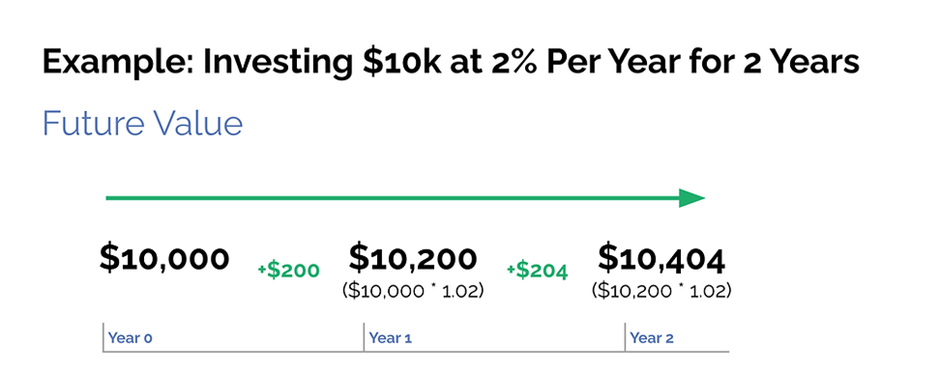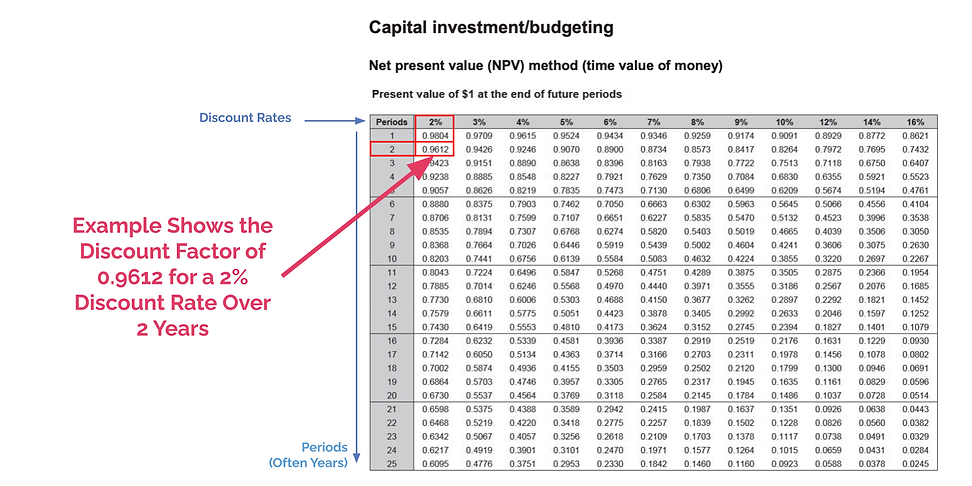top of page#### Time Value of Money

Content WritersChristian Bien

# What is Time Value of Money?

If someone offered you \$10,000 today or \$10,000 in 2 years time, which one would you choose? Of course most people would choose the \$10,000 today, but why?

The simple answer is that the value of \$10,000 today does not have the same value of \$10,000 in 2 years time.

For example, if a bank account offered an interest rate of 2%, if you invested that \$10,000 in two years you would have \$10,404. (Year 1 = 1.02 * \$10,000 = \$10,200. Year 2: 1.02 * \$10,200 = \$10,404.) Time Value is the same concept, just in reverse - instead of asking what is the value of an investment two years down the track, it is how much is it worth now if I receive it two years later?

# What is Future Value? - Example Investing \$10kFuture Value is simply the monetary value in a future point in time.

For example, if we invest \$10,000 at 2% per year, interest compounded annually, the future value would be \$10,404. Year 1 = 1.02 * \$10,000 = \$10,200. Year 2: 1.02 * \$10,200 = \$10,404.

# What is Present Value? - Example Investing \$10kTime value of money simply measures the reverse of the previous example. If we receive \$10,000 in two years time, and the current interest rate is 2%, the value of the money is divided to present day.

Year 2 = \$10,000

Year 1 = \$10,000 / 1.02 = \$9,803.92

Year 0 (Present Day) = \$9,803.92 / 1.02 = \$9,611.69

# What is the Discount Rate?

The discount rate refers to the percentage of which we reduce an amount by to achieve a present day valuation. The discount rate is subjective and can consist of a variety of factors, such as risk profile of the investment, inflation or current interest rates.

For questions in your exam, your discount rate will always be provided.

# How Do I Use the Annuity Table in the Specification Booklet?The annuity table is provided by SCSA to provide a simple way to discount. The top table is for finding the discounted value where this is only one cash inflow. Remember the example above with the 2% annual rate over 2 years.

We can see in the top table, under 2% for 2 periods, the result is 0.9612. If we multiply \$10,000 by 0.9612, we achieve the same result as above. = \$10,000 * 0.9612 = \$9,612. The bottom table is for multiple cash inflows, such as, if we received \$10,000 per year.

The bottom tables are just accumulated tables of the above.

Image of Specification Booklet Provided SCSA WA.